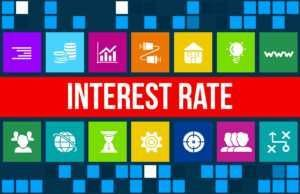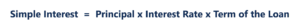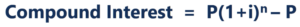# Interest Rate

Amount charged by a lender to a borrower for a debt

## What is an Interest Rate?

An interest rate refers to the amount charged by a lender to a borrower for any form of debt given, generally expressed as a percentage of the principal. The asset borrowed can be in the form of cash, large assets such as vehicle or building, or just consumer goods. In the case of larger assets, the interest rate is commonly referred to as “lease rate.”Interest rates are directly proportional to the amount of risk associated with the borrower. Interest is charged as compensation for the loss caused to the asset due to use. In the case of lending money, the lender could’ve invested the money in some other venture instead of giving it as a loan. In the case of lending assets, the lender could’ve generated income by making use of the asset himself. Thus, in return for these lost opportunities, interest rates are applied as compensation.

Annual interest rate refers to the rate that is applied over a period of one year. Interest rates can be applied over different periods, such as monthly, quarterly, or bi-annually. However, in most cases interest rates are annualized.

Interest rate can also refer to the rate paid by the bank to its clients for keeping deposits in the bank.

### Fixed vs. Floating (Variable) Rate

Interest rates can be fixed, where the rate remains constant throughout the term of the loan, or floating, where the rate is variable and can fluctuate based on a reference rate. More information regarding these two types of loan features can be found in the following article: Loan Features.

### Cost of Borrowing

The interest expense that is also known as the cost of borrowing money can be classified into the following two types:

#### #1 Simple Interest

This type of interest is calculated on the original or principal amount of loan. The formula for calculating simple interest is:For example, if the simple interest rate is given to be 5% on a loan of \$1,000 for a duration of 4 years, the total simple interest will come out to be: 5% x \$1,000 x 4 = \$200.

#### #2 Compound Interest

Compound interest is not just calculated on the basis of the principal amount but also on the accumulated interest of previous periods. This is the reason why it is also called “interest on interest.” The formula for compound interest is as follows:Where:

• P = Principal amount
• i = Annual interest rate
• n = Number of compounding periods for a year

Unlike simple interest, the compound interest amount will not be the same for all years because it takes into consideration the accumulated interest of previous periods as well.

### Real and Nominal Interest Rates

A nominal interest rate is one with no adjustments made for inflation. In other words, regardless of the rate of inflation in the economy, the interest received, for example, on a deposit, will be the same even after a given number of years.

A real interest rate takes the inflation rate into account. The repayment of principal plus the interest is measured on the basis of real terms compared against the buying power of the amount at the time it was borrowed, lent, invested, or deposited.

It’s important to factor in the effects of inflation on purchasing power because that’s the only way to know if you’re really earning a return from the interest being paid. For example, if you deposit money with a bank and earn a nominal 2% annual interest – if the inflation rate is 4%, then in terms of purchasing power, the money you have on deposit is actually losing 2% of its value every year. The real rate of return on an interest-bearing account is the nominal interest rate MINUS the rate of inflation. The stated interest rate is just the “nominal” rate, meaning “in name only” – i.e., not the REAL rate being earned.

### Factors Affecting Interest Rates

#### 1. Forces of demand and supply

Interest rates are influenced by the demand for, and supply of, credit in an economy. An increase in demand for credit eventually leads to a rise in interest rates, or the price of borrowing. Conversely, a rise in the supply of credit leads to a decline in interest rates. The credit supply increases when the total amount of money that’s borrowed goes up.

For example, when money is deposited in banks, it is in turn used by banks for investment activities or to lend it elsewhere. As banks lend more money, there is more credit available, and thus borrowing increases. When this occurs, the cost of borrowing decreases (due to normal supply and demand economics).

#### 2. Inflation

The higher the inflation rate, the higher interest rates rise. That is because interest earned on money loaned must compensate for inflation. As compensation for a decline in the purchasing power of money that they will be repaid in the future, lenders charge higher interest rates.

#### 3. Government

In some cases, the government’s monetary policy influences the amount of interest rates. Also, when the government buys more securities, banks are injected with more money to be used for lending, and thus interest rates decrease. When the government sells these securities, money from the banks gets drained, giving banks less money for lending purposes and leading to a rise in interest rates.

Thank you for reading this guide to interest rates. To continue advancing your career as a Financial Modeling and Valuation Analyst (FMVA)®, these additional resources will be helpful:

• Cost of Debt
• Coupon Rate
• Law of Supply
• Transfer Pricing

### Financial Analyst Training

Get world-class financial training with CFI’s online certified financial analyst training program!

Gain the confidence you need to move up the ladder in a high powered corporate finance career path.

Learn financial modeling and valuation in Excel the easy way, with step-by-step training.